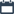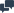## Tuesday, October 31, 2017

•4:26 PM
••No comments

## How the caution orders protect the rail track

How the caution orders protect the rail track and ensure the safe passing of trains over caution spot ---

While locomotive / train runs on track it puts a force on rails which reaches to ground through fully fit resilient pads , sleepers and ballast . In case anyone or more not fully fit to transfer the load to ground then by three ways train can pass safely over the unfit / less fit portion of track ----

1 by decreasing the load on axle or

2 by decreasing the speed of train or

3 by increasing the radius of wheels.

As we understand that the option 1 and 3 is not possible in a running train so the 2nd option is adopted for safe movement of trains over caution spot.

This force is calculated by the formula which is

F = M × V × V ÷ R

where

F is force act on rail by the running train is in ( Newton )

M is total mass on axle in kg

V is velocity of train in m/s

R is radius of wheel of locomotive.

For example

If a locomotive whose axle load is 20 ton and wheel radius is 0.5 m is running on the track with or without train at a speed of 110 kmph then the force on rail by per wheel of the locomotive will be

Given axle load is 20 ton then load on a wheel is 10 ton and then mass will be 10000 ÷ 10 = 1000 kg.

Given speed is 110 kmph means 110 ÷ 3.6 = 30.5 m/s  And radius is 0.5 m  then the force will be

F = 1000 × 30.5 × 30.5 ÷ 0.5

= 305 × 305 × 5

= 465125 N or 465.125 KN.

the above is the force put on rail by per wheel.

Now we how the reduced speed reduce force for safe passing

At 75 kmph

F = 1000 × 20.8 ×20.8 ÷ 0.5

= 208 × 208 × 5

= 216320 N or 216.320 KN

At 50 kmph

F = 1000 × 13.8 × 13.8 × 0.5

= 138 ×138 × 5

= 95220 N or 95.22 KN

At 30 kmph

F = 1000 × 8.3 × 8.3 × 0.5

= 83 × 83 × 5

= 34445 N or 34.445 KN.

At 20 kmph

F = 1000 × 5.5 × 5.5 × 0.5

= 55 × 55 × 5

= 15125 N or 15.125 KN

At 10 kmph

F = 1000 × 2.77 × 2.77 × 0.5

= 27.7 × 27.7 × 5

= 3836 N or 3.836 KN.

At stop dead formula changes

F = W = m × g = 1000 × 9.81

= 9810 N or 9.81 KN.

The above values are not accurately but approximately because both rails are not vertical but bent inside by 15 degree from vertical and wheel profile is also not flat but tapered.

Sleepers are provided so nearer to ensure that only one wheel should be on rail above one sleeper or on rail between two sleepers at a time.

Rail vehicles with different loads and different wheel radius put different forces at same speed.

The main purpose here is to know the requirement and role of caution orders.

## Blog Archive

AILRSA 1970 - . Powered by Blogger.

## -------------------------------------------------------